# 5 classical manifolds obtained by gluing edges of the square.

in #mathematicslast year

This post will have lower quality than my last post, but better such than none.

Imagine You have a square: [-1;1]x[-1;1] on the Euclidean plane R^2.

We can "glue" edges of that square by defining an equivalence relation. Here are 5 classical attempts:

1. (−1, t) ∼R1 (1, t)
2. (−1, t) ∼R2 (1, −t)
3. (−1, t) ∼R3 (1, t) and (t, −1) ∼R3 (t, 1)
4. (−1, −t) ∼R4 (1, t) and (t, −1) ∼R4 (t, 1)
5. (−1, −t) ∼R5 (1, t) and (−t, −1) ∼R5 (t, 1)

In every case we leave the points inside a circle unglued.

Of course You can define 2^2^Continuum relations on that square. But, these are interesting because they respond to gluing edges by "good" lines, while intern points are left as they were.

What does the first relation means?
That "left" edge of the square is identified with right edge of the circle. So our new object does not have left edge nor right edge.
What do we obtain?

Lateral surface of the cylinder.

Unfortunately, I'm too weak at programming at that moment to program myself these surfaces. Images are from Google :(: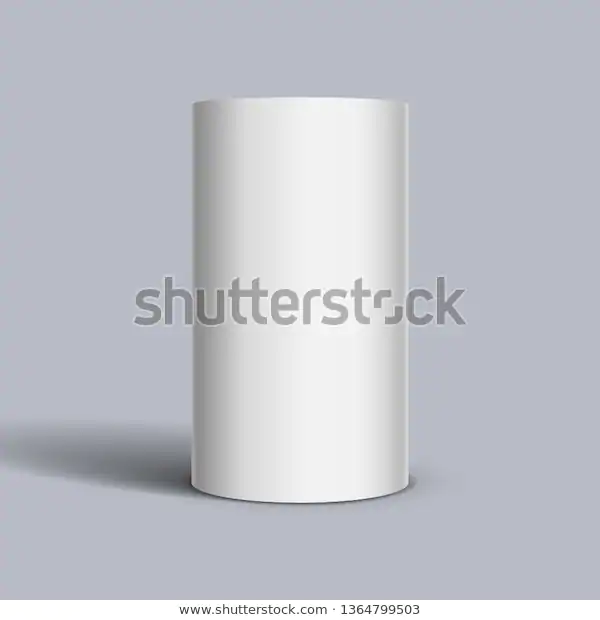Second relation:

Imagine You keep two edges of the square. You rotate one edge around its center by 180degree. Than, You glue like in first relation.
We obtain a Mobius strip: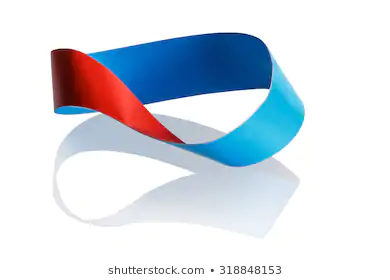Third relation:

We glue left edge with right edge (so we obtain lateral surface of the cylinder like in 1)) and then we glue top edge with bottom edge.
What do we obtain?
Torus, or like American policemen would say: donut.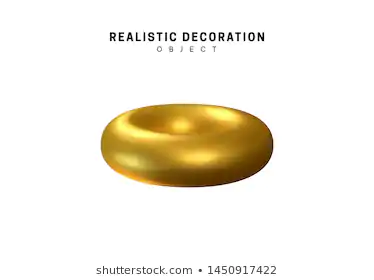(Look that we could obtain hotizontal donut or vertical donut, depending on what pair of edges we glued first, assuming we dont move doing gluing).

Last two examples are harder.
Fourth is real projective plane.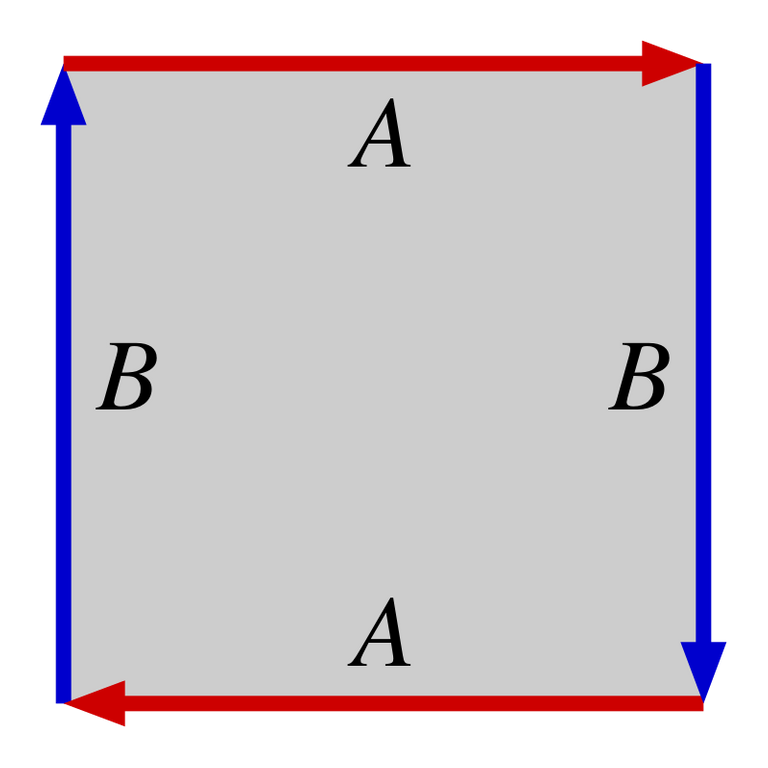You can't glue that in three dimensional space which has limitations.
You can glue it in four dimensional space.
Or, You can represent our object even in two dimensions, but in such representation we think only about relation, not about gluing.
You have to think that two points on the border, which are symmetrric by (0,0) are THE SAME point.
Moreover, You can even think of it as a circle with identified points symmetric with respect to (0,0) on the border.

Here is beautiful graphic: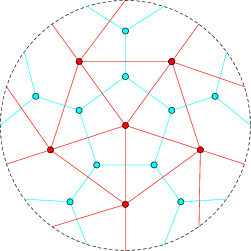The red and blue graphs are drawn properly - because antipodic points on the circle are the same point.

And the fifth relation corresponds to Klein bottle, which is totally abstract.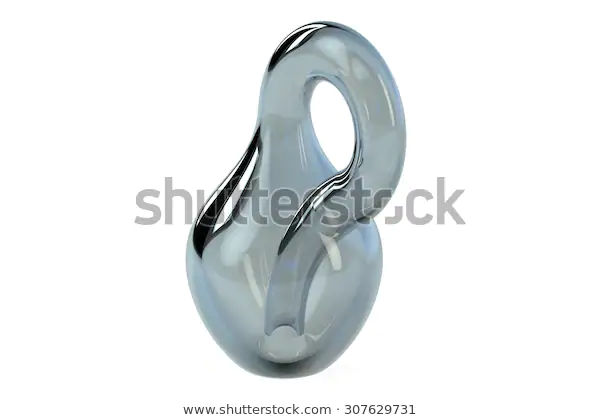This is also impossible to glue in three dimension, but can be done in four.
Klein bottle is a Mobius strip glued as a cylinder.

Thanks for watching that post.